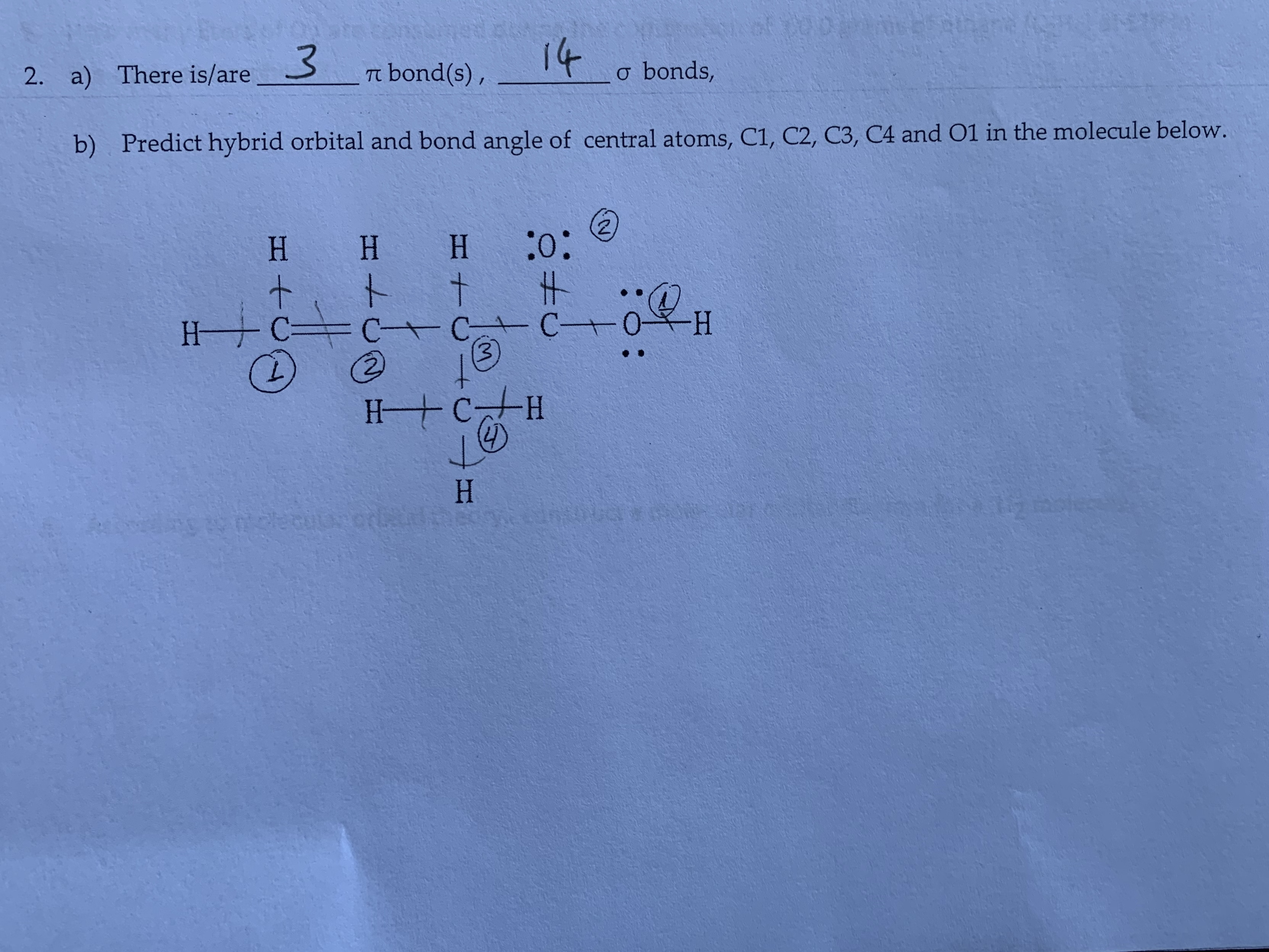# t2001OD32. a) There is/arebond(s),o bonds,b)Predict hybrid orbital and bond angle of central atoms, C1, C2, C3, C4 and O1 in the molecule below.HH H+tI#C C C3CHH CHH2HS0ceaoppor

Question
97 viewshelp_outlineImage Transcriptioncloset 2001 OD 3 2. a) There is/are bond(s), o bonds, b) Predict hybrid orbital and bond angle of central atoms, C1, C2, C3, C4 and O1 in the molecule below. H H H + t I# C C C 3 C H H CH H 2H S0ceaoppor fullscreen
check_circle

Step 1

The sigma bond is formed by the head to head overlapping of orbitals and the pi-bond is formed by the sideways overlapping of orbitals.

Step 2

Part (a)

In an organic compound, in triple bond one bond is sigma and two bonds are pi-bonds. In double bond, one bond is sigma and one is pi-bond. In single bond, bond is sigma bond.

In the given compound, there are 2 pi bonds and 14 sigma bonds as shown below.

Step 3

Part (b)

In the given compound, the C1 is surrounded by three electron groups. Therefore, the hybridization of C1 is sp2 and the geometry around C1 is trigonal planar. Hence, the bond angle around C1 is 120o.

In the given compound, the C2 is surrounded by three electron groups. Therefore, the hybridization of C2 is sp2 and the geometry around C2 is trigonal planar. Hence, the bond angle around C2 is 120o.

In the given compound, the C3 is surrounded by four electron groups. Therefore, the hybridization of C3 is sp3 and the geometry around C3 is tetrahedral. Hence, the bond angle around C3 is 109.5o.

In the given compound, the C4 is surrounded by four electron groups. Therefore, the hybridization of C4 is sp3 and the geometry around C4 is tetrahedral. Hence, the bond angle around C4 is...

### Want to see the full answer?

See Solution

#### Want to see this answer and more?

Solutions are written by subject experts who are available 24/7. Questions are typically answered within 1 hour.*

See Solution
*Response times may vary by subject and question.
Tagged in

### Organic Chemistry